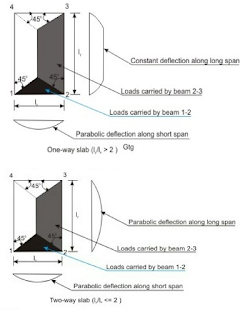# Difference Between One Way Slab and Two Way SlabONE WAY SLAB:
While designing a slab, if bending is considered to be occurring in one direction only then it is called a one-way slab. To be more clear see the diagram, below.

#### Conditions which holds good for one-way slab:

If a slab is supported by beams on two opposite sides, no matter what the dimensions are, the slab is designed as a one-way slab.
If the slab is supported by beams on all four sides, then we check the ratio of longer span(Lx) to shorter span(Ly). If this value is 2 then the slab is designed as a one-way slab.
Verandah slab is one example of a one-way slab.

### TWO WAY SLAB:

Similarly, if bending is considered to be occurring in both directions then it is called two-way slab. The diagram below illustrates it more clearly.

#### Conditions to identify two-way slab:

If the slab is supported by beams on all four sides and the ratio of longer span(Lx) to shorter span(Ly) is less than 2, then the slab is designed as a two-way slab.

### DIFFERENCE BETWEEN ONE WAy AND TWO WAY SLABS:

Before concluding let's summarize basic points that justify the difference Between these two types of slabs.
1. In one way slab, a major portion of loads is carried along one direction. Whereas in two-way slab load is carried along with both the directions. See the figure below.Load sharing in slabs
1. The (Lx /Ly) ratio is more than or equals to 2 in the one-way slab, whereas (Lx /Ly) is less than 2 in the two-way slab.